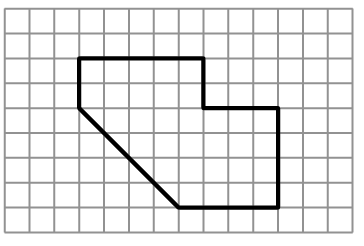### Home > INT2 > Chapter 8 > Lesson 8.3.1 > Problem8-57

8-57.Examine the shape at right.

1. What are the area and perimeter of the shape?

Use the graph to determine the lengths. Remember to draw in right triangles and use the Pythagorean Theorem to determine the length of the diagonal segment.

Perimeter $\approx25.7$ units

2. On graph paper, enlarge the figure so that the linear scale factor is $3$. What are the area and perimeter of the new shape?

Multiply each of the dimensions of this figure by $3$ to draw the new, larger figure.

Perimeter $\approx77$ units

3. What is the ratio of the perimeters of the shapes? What is the ratio of the areas?

The ratio of the perimeters is not the same as the ratio of the areas.